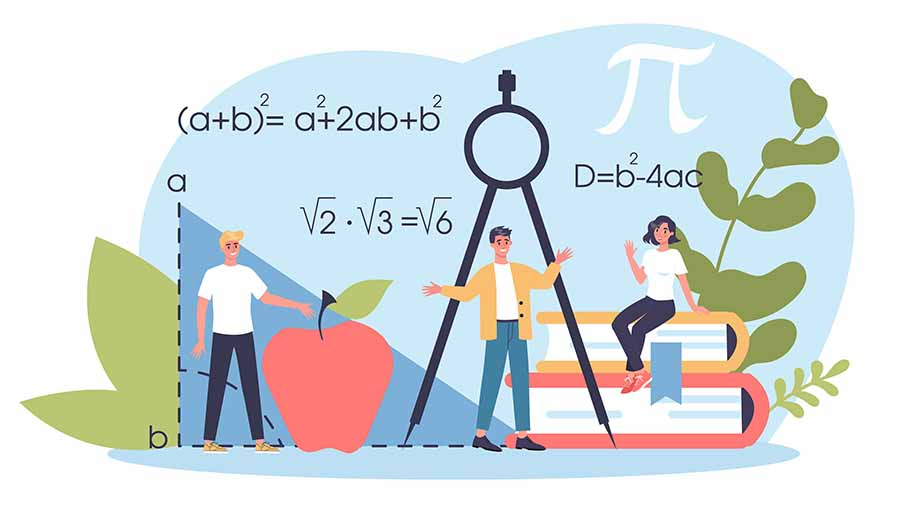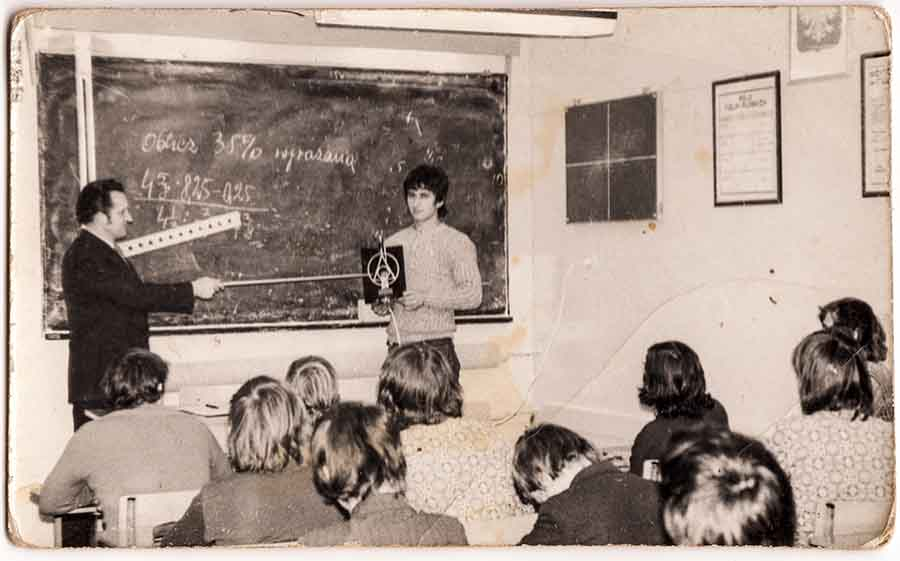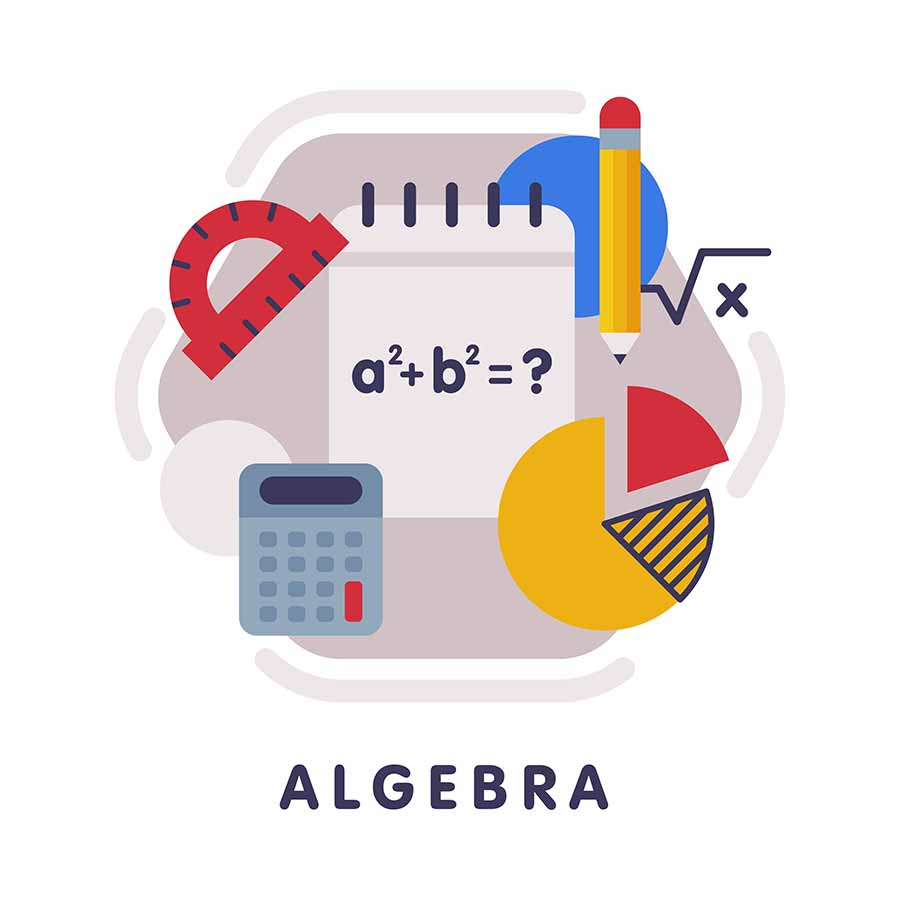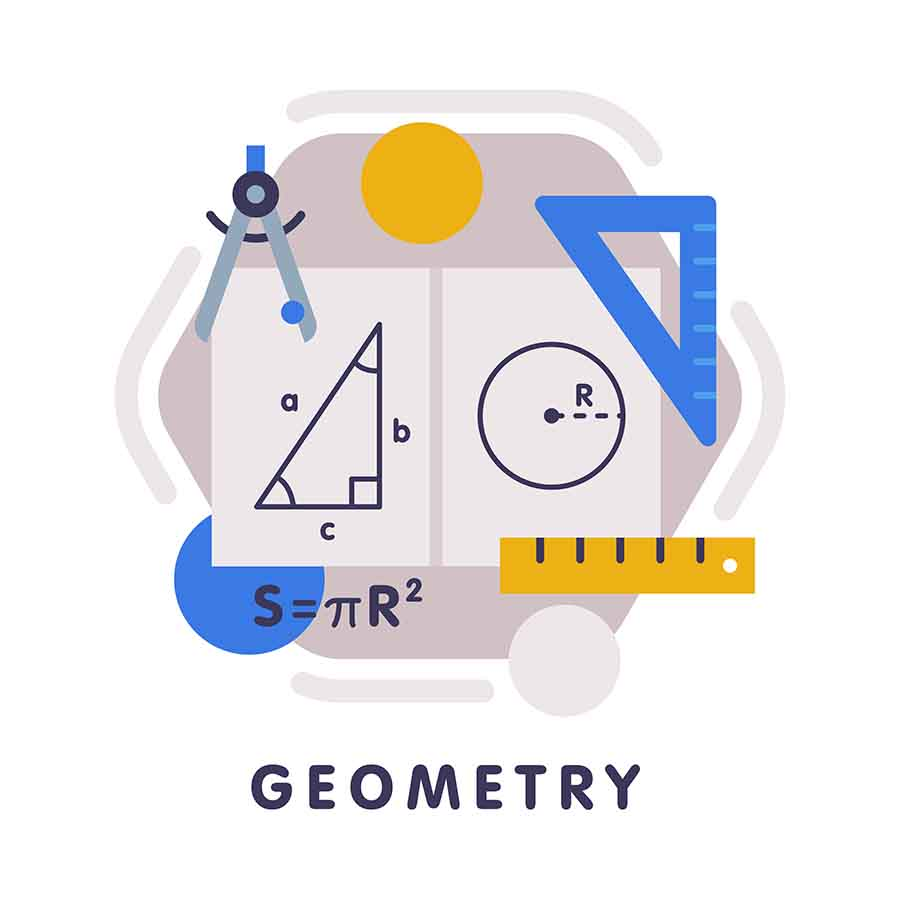## 02 Aug Introducing The Branches Of MathematicsMathematics is a science that has existed for millenniums. A building block for nearly everything in everyday life, it deals with the logic of structure, quantity and relation. Several different theories were formed in its early days, each contributing massively to their civilisation. In fact, the complexity of the math theory often correlated with the advancement in society.

A good reason for this relationship lies in that the more complex society is, the more complex its mathematical needs are. From this statement, we can see that mathematics is critical to solving issues or innovating solutions for society.

Many of the early mathematical theorems have evolved into the current branches of mathematics. Be it art, finances, construction, sports or travel, these branches of maths are applicable in all facets of life.

In this article, we will be looking at the beginning of mathematics and how their subsequent evolution into various applied forms of mathematics.

## The Beginning of MathematicsIt is difficult to pinpoint any single point in history for the birth of mathematics. This is because several civilizations have made major contributions to different components of mathematics. Early civilizations such as China, India, Persia, Egypt, Greece, Mayan and Mesopotamia have records of fundamental theorems that are used up till today.

Examples of early uses of mathematics include that of the Mayans who used maths to determine the time of the day and develop calendar systems. Meanwhile, Persian mathematicians would take the basic concepts of geometry and algebra in order to develop algorithms.

### Evolution of Mathematics

As alluded to before, an increasingly globalised world brought about an accelerated evolution of mathematics. Many new subject areas have evolved from its early base such as computational science and applied mathematics. These new subject paths along with the traditional tenants of mathematics have been classified into various branches of maths.

## Traditional Branches of Pure Mathematics

Pure mathematics can be defined as the basic concepts that underlie all forms of maths. It is called “pure” as its concepts are independent of any application outside of mathematics.

This is not to say that these concepts are not useful. Far from that, pure mathematics concepts have originated from real world concerns. Additionally, their findings have later being applied for practical uses.

However, mathematicians within pure mathematics fields are primarily interested in the deeper understanding and expanding of the knowledge of maths itself. As such, they are typically not motivated or concerned by the application of the concepts or theories.

### Arithmetic

Arithmetic is considered to be the most elementary branch of mathematics. Being the study of numbers, it includes the most basic operations of maths such as addition, subtraction, multiplication and division. Further advancements in arithmetic include the invention of the number “0” and decimals.

Students’ first encounter with mathematics in education usually comes through arithmetic. For instance, learning to count. Further along their education journey, they will be challenged by arithmetic concepts such as natural numbers, integers, fractions and decimals.

### AlgebraAlgebra is arguably one of the most utilised branches of pure mathematics. It deals with symbols and rules that help to simplify complex relationships and resolve them. The idea behind algebra is that symbols can be used to represent quantities without fixed values. Meanwhile its equations help to describe and determine the relationship between these values.

A common algebra equation is that of the linear equation. The linear equation’s general form is “y = mx + c”. When mapped out on a graph with values inserted, a straight line will always be formed. This also means that were you know 3 possible values in the equation, you can definitely determine the fourth.

### GeometryGeometry is a highly visible branch of pure mathematics. It concerns the shapes and sizes of figures, coupled with their properties. Be it lines, angles, surfaces or volumes, Geometry helps us to in many real-life decisions.

Intuitively, we do have spatial understanding and visual comprehension of our surroundings. For instance, we can describe the shape of an object or recognise when something comes close to us. Geometry is then simply put a detailed study into our understanding of space and shapes around us. Through geometry, we are able to better describe and comprehend everyday objects.

Examples of the application of geometry include:

• Calculating how much water you need in order to fill a bottle
• Designing leisure kites to be flown
• Determining the size of support pillars for a building

### CalculusCalculus is a relatively less visible form of mathematics. Afterall it is the study of continuous change and is known as the “calculus of infinitesimals”. There are 2 primary categories of calculus – differential calculus and integral calculus.

Differential calculus deals with instantaneous rates of change such as the slope of curves. This allows you to use differentiation to analyse and predict a graph. Meanwhile, integral calculus is concerned with accumulation of quantities such as the area under a curve. In contrast, under this category, integration is used to reverse differentiation.

When used together, these 2 derivatives can predict how a graph may look and its trend or shape. Real world applications of calculus are thus plenty, being fundamental to fields such as physics, engineering and economics. Imagine calculating the rate of falloff from a ball’s trajectory or computing the marginal revenue in economics.

## Application Branches of Mathematics

Other than pure mathematics, there are mathematicians and related professions that look to work on mathematics from an applied point of view. Oftentimes, these fields use pure mathematics branches as a base while also incorporating more real world issues.

### Cartesian Geometry

Cartesian geometry is the study of geometry using a coordinate system. Simply put, by mapping points in a coordinate system, you would be able to determine the shape of an object or amount of space within it. Also known as analytical geometry, it is most famously used in physics, engineering, aviation and space science.

### Matrix Algebra

Matrix algebra uses an array table to display algebra variables. In doing so, large expressions and relationships can be expressed in an efficient manner.

### Other forms of Applied Mathematics

• Topology
• Analysis
• Combinatorics
• Order theory
• Discrete maths

This is list is by no means exhaustive and serves demonstrate how mathematics has evolved to include a variety of fields.

## Trust MLGS to Improve Your Child’s Maths

Whatever the reason, signing your child up for maths tuition is a great way to ensure that they are getting the help they need. If you are looking for a primary math tuition centre Singapore service, Maths Learning Groups may just be the right one for you.

Established by ex-primary school teacher Mr Lawrence Ong, we are a Clementi primary maths tuition centre that aims to uplift students who struggle with maths, by identifying their individual weaknesses and developing teaching plans that are tailored to their individual learning styles. We are committed to helping students improve by strengthening their foundation in key maths concepts, and setting them up for the future by instilling crucial skills like analytical reasoning and critical thinking.